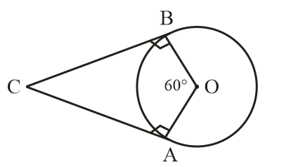#### Write ‘True’ or ‘False’ and justify your answer in each of the following : If a chord AB subtends an angle of  at the centre of a circle, then angle between the tangents at A and B is also .SolutionHere CA and CB are the two tangents which is drawn on chord AB and also we know that tangent and radius are perpendicular to each other.
i.e.,

[  Sum of interior angles of a quadrilateral is ]

Here we conclude that angle between the tangents at A and B is .

Therefore the given statement is False.